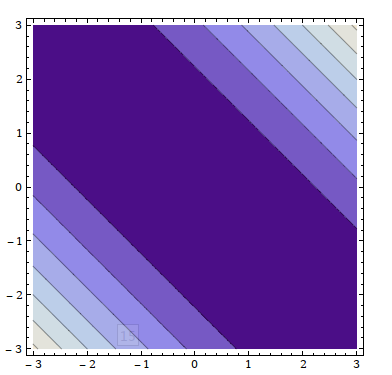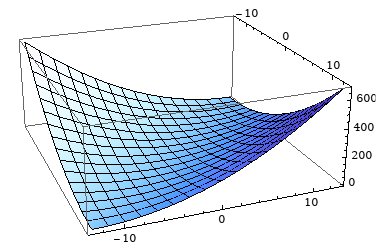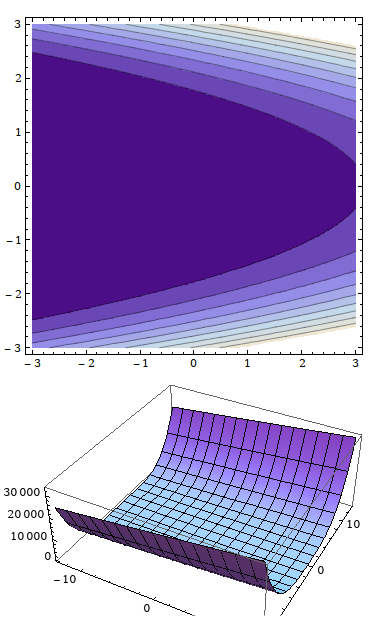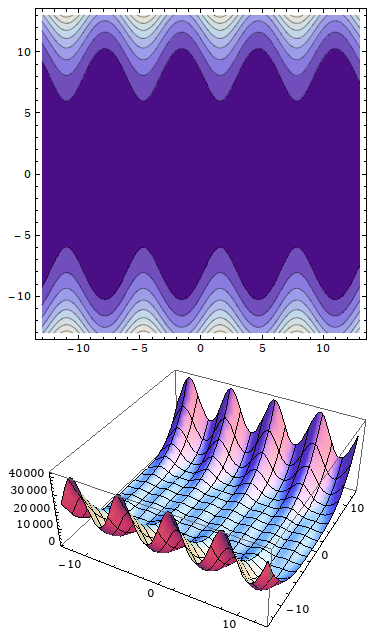## Change of variables and degeneration

Consider the functin $f(x, y)$, e.g:

\begin{align*} f(x, y) &= (x+y)^2 \\ f(x, y) &= (x+y^2)^2 \\ f(x, y) &= (35 \sin x+y^2)^2 \\ \end{align*}Dennis Auroux called this kind of functions "degenerate" because then can be written as $f(u(x, y))$: https://www.youtube.com/watch?v=3_goGnJm5sA

I am not sure what this is suppose to mean, could anyone explain?